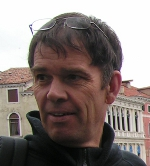###Contact

Luc Biard
Laboratoire Jean Kuntzmann (LJK) - Bâtiment IMAG
Université Grenoble Alpes
700 Avenue Centrale
38401 Domaine Universitaire de Saint-Martin-d'Hères (France)
Luc.Biard@univ-grenoble-alpes.fr

Phone : +33 4 57 42 17 08Professor at  UGA
Researcher at Laboratoire Jean Kuntzmann ( LJK ) CAGD team  CVGICV Research Projects TeachingShort CVEnglish (pdf)hdr (pdf)Research interestsRecent PublicationsLJK-bibliographyHAL-bibliographyMSTIC - GeoNorEquipe de Recherche Commune      CEA-Leti / UJF-LJK      Géométrie et Capteurs Being assessedMiCaGeo (blanc ANR)Myanmar collaboration         HereLicence 1 : Map110-120         Documents iciLicence 2 : Mat234         Programme    DLST         Documents iciL3 MI : Algèbre linéaire         pour le Graphique et la CAO         HereM1 MAI : Géométrie Appliquée         Programme    MAI         Documents ici##Curriculum Vitae

 1990 Ph.D. Thesis, Université Joseph Fourier - Grenoble - Applied Mathematics 1991 Associate Professor at UJF (Université Joseph Fourier) - Grenoble (France) 2007-2008 Visiting Professor at UC Davis, USA 2009 Habilitation à Diriger des Recherches (Grenoble Université) 2014 Professor at UJF (Université Joseph Fourier) - Grenoble (France)##Research interests

Computer aided geometric design
Geometric models - developable surfaces
Spiral and Transition curves - PH curves
Bézier curves properties
Capture and reconstruction of curves and surfaces from tangential data
Computational geometry - shortest and geodesic paths
Top##Recent Publications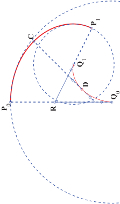Pythagorean hodograph spline spirals that match G3 Hermite data from circles Zhong Li, Rachid Ait-Haddou and Luc Biard Journal of Computational and Applied Mathematics, 278, pp. 162-180, 2015 Abstract : A construction is given for a G3 piecewise rational Pythagorean hodograph convex spiral which interpolates two G3 Hermite data associated with two non-concentric circles, one being inside the other. The spiral solution is of degree 7 and is the involute of a G2 convex curve, referred to as the evolute solution, with prescribed length, and composed of two PH quartic curves. Conditions for G3 continuous contact with circles are then studied and it turns out that an ordinary cusp at each end of the evolute solution is required. Thus, geometric properties of a family of PH polynomial quartics, allowing to generate such an ordinary cusp at one end, are studied. Finally, a constructive algorithm is described with illustrative examples.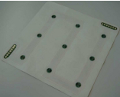Surfaces reconstruction via inertial sensors for monitoring Nathalie Saguin-Sprynski, Laurent Jouanet, Bernard Lacolle and Luc Biard 7th European Workshop on Structural Health Monitoring, pp. 702-709, 2014 Abstract : This document deals with the new capabilities of monitoring via the surface reconstruction of structures with sensors arrays systems. Indeed, we will detail here our new demonstrator composed of a smart textile equipped with inertial sensors and a set of processings allowing to reconstruct the shape of the textile moving along time. We show here how this new tool can provide very useful information from the structures.C2 interpolation of spatial data subject to arc-length constraints using Pythagoreanhodograph quintic splines Mathieu Huard, Rida T. Farouki, Nathalie Sprynski and Luc Biard Graphical Models, 76, pp. 30-42, 2014 Abstract : In order to reconstruct spatial curves from discrete electronic sensor data, two alternative C2 Pythagoreanhodograph (PH) quintic spline formulations are proposed, interpolating given spatial data subject to prescribed constraints on the arc length of each spline segment. The first approach is concerned with the interpolation of a sequence of points, while the second addresses the interpolation of derivatives only (without spatial localization). The special structure of PH curves allows the arc-length conditions to be expressed as algebraic constraints on the curve coefficients. The C2 PH quintic splines are thus defined through minimization of a quadratic function subject to quadratic constraints, and a close starting approximation to the desired solution is identified in order to facilitate efficient construction by iterative methods. The C2 PH spline constructions are illustrated by several computed examples.Reconstruction of quasi developable surfaces from ribbon curves Mathieu Huard, Nathalie Sprynski, Nicolas Szafran and Luc Biard Numerical Algorithm, 63, pp. 483-506, 2013 Abstract : This paper deals with the acquisition and reconstruction of physical surfaces by mean of a ribbon device equipped with micro-sensors, providing geodesic curves running on the surface. The whole process involves the reconstruction of these 3D ribbon curves together with their global treatment so as to produce a consistent network for the geodesic surface interpolation by filling methods based on triangular Coons-like approaches. However, the ribbon curves follow their own way, subdividing thus the surface into arbitrary n-sided patches. We present here a method for the reconstruction of quasi developable surfaces from such n-sided curvilinear boundary curves acquired with the ribbon device.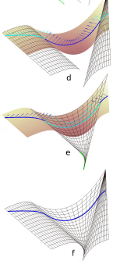Rubans géodésiques pour la segmentation et la reconstruction de surfaces développables Mathieu Huard, Nathalie Sprynski, Nicolas Szafran, Luc Biard Journées du GTMG, Strasbourg, Mars 2012 Abstract : This paper deals with the acquisition and reconstruction of physical developable G1 surfaces by using a ribbon equipped with micro-accelerometers and micro-magnetometers, providing geodesic curves running on the surface. The method consists in working with the space of tangent planes provided by the geodesics, where the different components of the surface can be identified, and then reconstructed as beams of lines going through the acquired geodesics.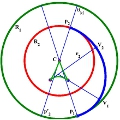On the interpolation of concentric curvature elements Carlotta Giannelli and Luc Biard Computer-Aided Design, Volume 43, Issue 6, pp. 586-597, June 2011 Abstract : A convex G2 Hermite interpolation problem of concentric curvature elements is considered in this paper. It is first proved that there is no spiral arc solution with turning angle less than or equal to $\pi$ and then, that any convex solution admits at least two vertices. The curvature and the evolute profiles of such an interpolant are analyzed. In particular, conditions for the existence of a G2 convex interpolant with prescribed extremal curvatures are given.Motion Capture of an Animated Surface via Sensors Ribbons Nathalie Sprynski, Bernard Lacolle and Luc Biard In Proceeding of the First International Conference on Pervasive and Embedded Computing and Communication Systems, Vilamoura, Algarve, Portugal, 5-7 March, 2011 Abstract : This paper deals with the motion capture of physical surfaces via a curve acquisition device. This device is a ribbon of sensors, named Ribbon Device, providing tangential measurements, allowing to reconstruct its 3D shape via an existing geometric method. We focus here on the problem of reconstructing animated surfaces, from a finite number of curves running on these surfaces, acquired with the Ribbon Device. This network of spatial curves is organized according a comb structure allowing to adjust these curves with respect to a reference curve, and then to develop a global C1 reconstruction method based on the mesh of ribbon curves together with interpolating transversal curves. Precisely, at each time position the surface is computed from the previous step by an updating process.A Construction of rational surface patches bounded by lines of curvature Luc Biard, Rida T. Farouki, Nicolas Szafran Computer Aided Geometric Design, Volume 27(5), pp. 359--371, 2010 Abstract : The fact that the Darboux frame is rotation--minimizing along lines of curvature of a smooth surface is invoked to construct rational surface patches whose boundary curves are lines of curvature. For given patch corner points and associated frames defining the surface normals and principal directions, the patch boundaries are constructed as quintic RRMF curves, i.e., spatial Pythagorean--hodograph (PH) curves that possess rational rotation--minimizing frames. The interior of the patch is then defined as a Coons interpolant, matching the boundary curves and their associated rotation--minimizing frames as surface Darboux frames. The surface patches are compatible with the standard rational B\'ezier/B--spline representations, and $G^1$ continuity between adjacent patches is easily achieved. Such patches are advantageous in surface design with more precise control over the surface curvature properties.Construction and smoothing of triangular Coons patches with geodesic boundary curves Rida T. Farouki, Nicolas Szafran, Luc Biard Computer Aided Geometric Design, Volume 27(4), pp. 301--312, 2010 Abstract : Given three regular space curves ${\bf r}_1(t)$, ${\bf r}_2(t)$, ${\bf r}_3(t)$ for $t\in[\,0,1\,]$ that define a curvilinear triangle, we consider the problem of constructing a triangular surface patch ${\bf R}(u_1,u_2,u_3)$ bounded by these three curves, such that they are geodesics of the constructed surface. Results from a prior study \citep{Farouki09} concerned with tensor--product patches are adapted to identify constraints on the given curves for the existence of such geodesic--bounded triangular surface patches. For curves satisfying these conditions, the patch is constructed by means of a cubically--blended triangular Coons interpolation scheme. A formulation of thin--plate spline energy in terms of barycentric coordinates with respect to a general domain triangle is also derived, and used to optimize the smoothness of the geodesic--bounded triangular surface patches.A Refinement of the Variation Diminishing Property of Bézier Curves Rachid Ait-Haddou, Taishin Nomura and Luc Biard Computer Aided Geometric Design, Volume 27, Issue 2, Pages 202-211, February 2010 Abstract : For a given polynomial $F(t)= \sum_{i=0}^{n} p_i B_i^n(t)$, expressed in the Bernstein basis over an interval $[a,b]$, we prove that the number of real roots of $F(t)$ in $[a,b]$, counting multiplicities, does not exceed the sum of the number of real roots in $[a,b]$ of the polynomial $G(t)= \sum_{i=k}^l p_i B_{i-k}^{l-k}(t)$ (counting multiplicities) with the number of sign changes in the two sequences $(p_0,...,p_k)$ and $(p_l,...,p_n)$ for any value $k,l$ with $0\leq k \leq l \leq n$. As a by product of this result, we give new refinements of the classical variation diminishing property of B\'ezier curves.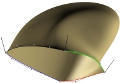Construction of Bézier surface patches with Bézier curves as geodesic boundaries Rida T. Farouki, Nicolas Szafran, Luc Biard Computer Aided Design, Vol. 41, pp. 772--781, 2009 Abstract : Given four polynomial or rational B\'ezier curves defining a curvilinear rectangle, we consider the problem of constructing polynomial or rational tensor--product B\'ezier patches bounded by these curves, such that they are geodesics of the constructed surface. The existence conditions and interpolation scheme, developed in a general context in earlier studies, are adapted herein to ensure that the geodesic--bounded surface patches are compatible with the usual polynomial/rational representation schemes of CAD systems. Precise conditions for four B\'ezier curves to constitute geodesic boundaries of a polynomial or rational surface patch are identified, and an interpolation scheme for the construction of such surfaces is presented when these conditions are satisfied. The method is illustrated with several computed examples.Existence conditions for Coons patches interpolating geodesic boundary curves Rida T. Farouki, Nicolas Szafran, Luc Biard Computer Aided Geometric Design, Volume 26, Issue 5, pp. 599-614, June 2009 Abstract : Given two pairs of regular space curves ${\bf r}_1(u)$, ${\bf r}_3(u)$ and ${\bf r}_2(v)$, ${\bf r}_4(v)$ that define a curvilinear rectangle, we consider the problem of constructing a $C^2$ surface patch ${\bf R} (u,v)$ for which these four boundary curves correspond to geodesics of the surface. The possibility of constructing such a surface patch is shown to depend on the given boundary curves satisfying two types of consistency constraints. The first constraint is global in nature, and is concerned with compatibility of the variation of the principal normals along the four curves with the normal to an oriented surface. The second constraint is a local differential condition, relating the curvatures and torsions of the curves meeting at each of the four patch corners to the angle between those curves. For curves satisfying these constraints, the surface patch is constructed using a bicubically--blended Coons interpolation process.Pythagorean-hodograph ovals of constant width Ait-Haddou Rachid, Walter Herzog and Luc Biard Computer Aided Geometric Design, Volume 25, 4-5, pp. 258--273, May 2008 Abstract : A constructive geometric approach to rational ovals and rosettes of constant width formed by piecewise rational PH curves is presented. We propose two main constructions. The first construction, models with rational PH curves of algebraic class $3$ (T-quartics) and is based on the fact that T-quartics are exactly the involutes of T-cubic curves. The second construction, models with rational PH curves of algebraic class $m > 4$ and is based on the dual control structure of offsets of rational PH curves.Surface reconstruction via geodesic interpolation Nathalie Sprynski, Nicolas Szafran, Bernard Lacolle and Luc Biard Computer-Aided Design, Volume 40, Issue 4, pp. 480-492, April 2008 Abstract : This paper is concerned with reconstruction of numerical or real surfaces based on the knowledge of some geodesic curves on the surface. So, considering two regular 3D-curves $f_0(t)$ and $f_1(t)$, our purpose is to construct a surface which interpolates these two curves in such a way that these two curves are geodesics on this surface. This will be accomplished using Hermite interpolation. For a real surface, it will be shown that geodesics can be acquired using a ribbon of micro-sensors.Curve and Surface Reconstruction via Tangential Information Nathalie Sprynski, Bernard Lacolle, Dominique David and Luc Biard Curve and Surface Design : Avignon 2006, P. Chenin, T. Lyche, L. L. Schumaker (eds), Nashboro Press, pp. 254--263, 2007 Abstract : This paper presents a novel method for recontructing surfaces relying only on tangential data which are provided by embedded sensors. The reconstruction process is based on the knowledge of the distribution of the sensors, which are organized as a square mesh, and on the associated tangential orientation measurements without any information about their positioning in space so that this problem cannot be solved by envelope methods. We provide methods for planar and spatial curves, then extend them for surfaces. We validate these methods proving their convergence and by the analysis of the results obtained in a physical point of view. Finally, we show the implementation of our work in a real time prototype.Curve Reconstruction via a Ribbon of Sensors Nathalie Sprynski, Bernard Lacolle, Dominique David and Luc Biard In Proceeding of the 14th IEEE International Conference on Electronics, Circuits and Systems, ICECS - 2007, December 2007 Abstract : This paper presents a novel method for reconstructing curves relying on tangential data which are provided by embedded sensors. The reconstruction process is based on the knowledge of the distribution of the sensors along the curve, represented by a ribbon, and on the associated tangential orientation measurements without any information about their positioning in space, so that this problem is not an envelope problem. We first show how we can obtain these data from sensors and the prototypes we have created. Then we provide methods for planar curves, then for spatial curves and we analyze results with physical sense and convergence in order to validate these methods. Finally, we show some results from both simulated data and real data.
Top##Teaching

 Map110 - Map120 : thème "Courbes" (L1) cours 1 (pdf) cours 2 (pdf) cours 3 (pdf) TP 1 (pdf) TP 2 (pdf) TP 3 (pdf) TP 4 (+ cours) (pdf) Mat234 (Licence 2) Résumé cours analyse (pdf) Résumé cours algèbre (pdf) Résumé cours algèbre V2 (pdf) Fiches TD (pdf) -- Corrigés TD analyse (pdf) -- Corrigés TD algèbre (pdf) Annales CC1 novembre 2009 (pdf) - Corrigé (pdf) CC2 décembre 2009 (pdf) - Corrigé (pdf) Examen1 décembre 2009 (pdf) Examen2 juin 2010 (pdf) CC1 novembre 2010 (pdf) - Corrigé (pdf) CC2 décembre 2010 (pdf) Examen1 janvier 2011 (pdf) Examen2 juin 2011 (pdf) CC1 novembre 2011 (pdf) - Corrigé (pdf) CC2 décembre 2011 (pdf) - Corrigé (pdf) Examen1 janvier 2012 (pdf) - Corrigé (pdf) Examen2 juin 2012 (pdf) CC1 octobre 2012 (pdf) CC2 décembre 2012 (pdf) - Corrigé (pdf) Examen1 janvier 2013 (pdf) - Corrigé (pdf) Examen2 juin 2013 (pdf) - Corrigé (pdf) CC1 novembre 2013 (pdf) - Corrigé (pdf) CC2 décembre 2013 (pdf) - Corrigé (pdf) Examen1 janvier 2014 (pdf) - Corrigé (pdf) Examen2 juin 2014 (pdf) - Corrigé (pdf) CC1 novembre 2014 (pdf) - Corrigé (pdf) CC2 décembre 2014 (pdf) - Corrigé (pdf) Examen1 janvier 2015 (pdf) - Corrigé (pdf) Examen2 juin 2015 (pdf) - Corrigé (pdf) CC1a octobre 2015 (pdf) - Corrigé (pdf) CC2 novembre 2015 (pdf) - Corrigé (pdf) CC1b décembre 2015 (pdf) - Corrigé (pdf) Examen1 janvier 2016 (pdf) - Corrigé (pdf) Algèbre linéaire pour le graphique et la CAO (L3 MI) Annales Examen décembre 2007 (pdf) - Examen session2 2007-08 (pdf) - Examen décembre 2008 (pdf) - Examen session2 2008-09 (pdf) - Examen décembre 2009 (pdf) - Examen décembre 2010 (pdf) - Examen décembre 2011 (pdf) - Examen décembre 2012 (pdf) - Examen décembre 2013 (pdf) - Partiel octobre 2014 (pdf) - DM novembre 2014 (pdf) - Examen décembre 2014 (pdf) - Partiel novembre 2015 (pdf) - Examen décembre 2015 (pdf) - Partiel octobre 2016 (pdf) - Examen décembre 2016 (pdf) - Partiel octobre 2017 (pdf) - Examen décembre 2017 (pdf) - Top Plan du cours Chap 01 : Intro Numpy et Matplotlib (pdf) Chap 02 : Pré-requis en maths Chap 03 : Interpolation de Lagrange --- Slides (pdf) Chap 04 : Interpolation de Hermite --- Slides (pdf) Chap 05 : Interpolation spline --- Slides (pdf) Chap 06 : Approximation aux moindres carrés Chap 07 : Approximation sous contraintes linéaires Chap 08 : Splines de lissage Chap 09 : Courbes et surfaces de Bézier TP 2022-2023 Fascicule des TPs (pdf) Scripts Python (squelettes) (zip) Indication pour TP3A (jpg) --- Liste des TPs : TP1A : Lists - Numpy  Matplotlib TP2A : Gauss  Conditioning  Gram-Schmidt & orthogonal matrices TP2B : Numerical integration & Fourier series TP3A : Interpolation de Lagrange 1D & 2D  base de Lagrange TP3B : Interpolation de Lagrange  base de Newton TP3C : Lagrange interpolation in monomial basis with scipy TP4 : Hermite interpolation TP5A : Interpolation spline cubique C2  cas explicite & paramétrique TP5B : Periodic interpolating cubic splines TP5C : Splines exponentielles sous tension TP6A : Approximation aux moindres carrées  Erreur résiduelle TP7 : Least squares approximation with integral constraint TP8 : Smoothing splines TP9A : Subdivision TP9B : Intersection de courbes de Bézier par Exclusion et Subdivision Projet Kinect Description (tasks / planning) pdf An interesting video (Kinect scanner tutorial, YouTube) here SDK Kinect pour Windows 2.0 here Meshlab here Skeleton scripts : [sur le drive] L3 student report [on the drive] Some mesh data from the web [on the drive] -------------------------------------------------------------------- Géométrie Appliquée (M1 MAI) TP1 : courbe Bézier - subdivision (pdf) TP2 : interpolation Bézier (pdf) TP3 : offset et evolute/involute (pdf) TP4 : principe d'exclusion (pdf) TP5 : spline cubique (pdf) TP6 : directions principales - Dupin (pdf) TP7 : deBoor-Cox (pdf) TP8 : insertion noeuds et subdivision spline (pdf) TP9 : spline périodique (pdf) TP10 : Bézier rationnelle (pdf) TP11 : courbe Nurbs (pdf) TP12 : surface Bézier tensorielle(pdf) TP13 : surface Bézier tensorielle rationnelle (pdf) TP14 : surface Nurbs (pdf)

TopLast update March 2012